Check the below Online Education NCERT MCQ Questions for Class 9 Maths Chapter 10 Circles with Answers Pdf free download. MCQ Questions for Class 9 Maths with Answers were prepared based on the latest exam pattern. We have provided Circles Class 10 Maths MCQs Questions with Answers to help students understand the concept very well. https://ncertmcq.com/mcq-questions-for-class-9-maths-with-answers/

Students can also refer to NCERT Solutions for Class 9 Maths Chapter 10 Circles for better exam preparation and score more marks.

## Circles Class 9 MCQs Questions with Answers

Circles Class 9 MCQ Chapter 10 Question 1.
The radius of a circle is 13 cm and the length of one of its chords is 10 cm. The distance of the chord from the centre is
(a) 11.5 cm
(b) 12 cm
(c) $$\sqrt{69}$$ cm
(d) 23 cm

MCQ On Circles Class 9 Chapter 10 Question 2.
A chord is at a distance of 8 cm from the centre of a circle of radius 17 cm. The length of the chord is
(a) 25 cm
(b) 12.5 cm
(c) 30 cm
(d) 9 cm

Circles MCQ Class 9 Chapter 10 Question 3.
In the given figure, BOC is a diameter of a circle and AB = AC. Then, ∠ABC =(a) 30°
(b) 45°
(c) 60°
(d) 90°

Circle Class 9 MCQ Chapter 10 Question 4.
In the given figure, O is the centre of a circle. If ∠OAB = 40° and C is a point on the circle, then ∠ACB =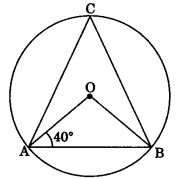(a) 40°
(b) 50°
(c) 80°
(d) 100°

Class 9 Circles MCQ Chapter 10 Question 5.
AB and CD are two equal chords of a circle with centre O such that ∠AOB = 80°, then ∠COD =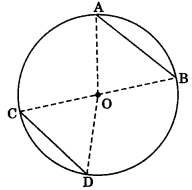(a) 100°
(b) 80°
(c) 120°
(d) 40°

Circle MCQ Class 9 Chapter 10 Question 6.
In the given figure, BOC is a diameter of a circle with centre O. If AB and CD are two chords such that AB || CD and AB = 10 cm, then CD =(a) 5 cm
(b) 12.5 cm
(c) 15 cm
(d) 10 cm

Class 9 Maths Chapter 10 MCQ Question 7.
In the given figure, AB is a chord of a circle with centre O and AB is produced to C such that BC = OB. Also, CO is joined and produced to meet the circle in D. If ∠ACD = 25°, then ∠AOD =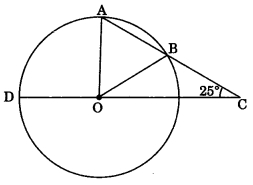(a) 50°
(b) 75°
(c) 90°
(d) 100°

MCQ Questions On Circles For Class 9 Question 8.
In the given figure, AB is a chord of a circle with centre O and BOC is a diameter. If OD ⊥ AB such that OD = 6 cm, then AC =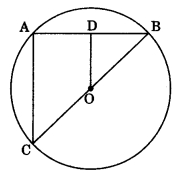(a) 9 cm
(b) 12 cm
(c) 15 cm
(d) 7.5 cm

Class 9 Maths Chapter 10 MCQ With Answers Question 9.
An equilateral triangle of side 9 cm is inscribed a circle. The radius of the circle is
(a) 3 cm
(b) 3√2 cm
(c) 3√3 cm
(d) 6 cm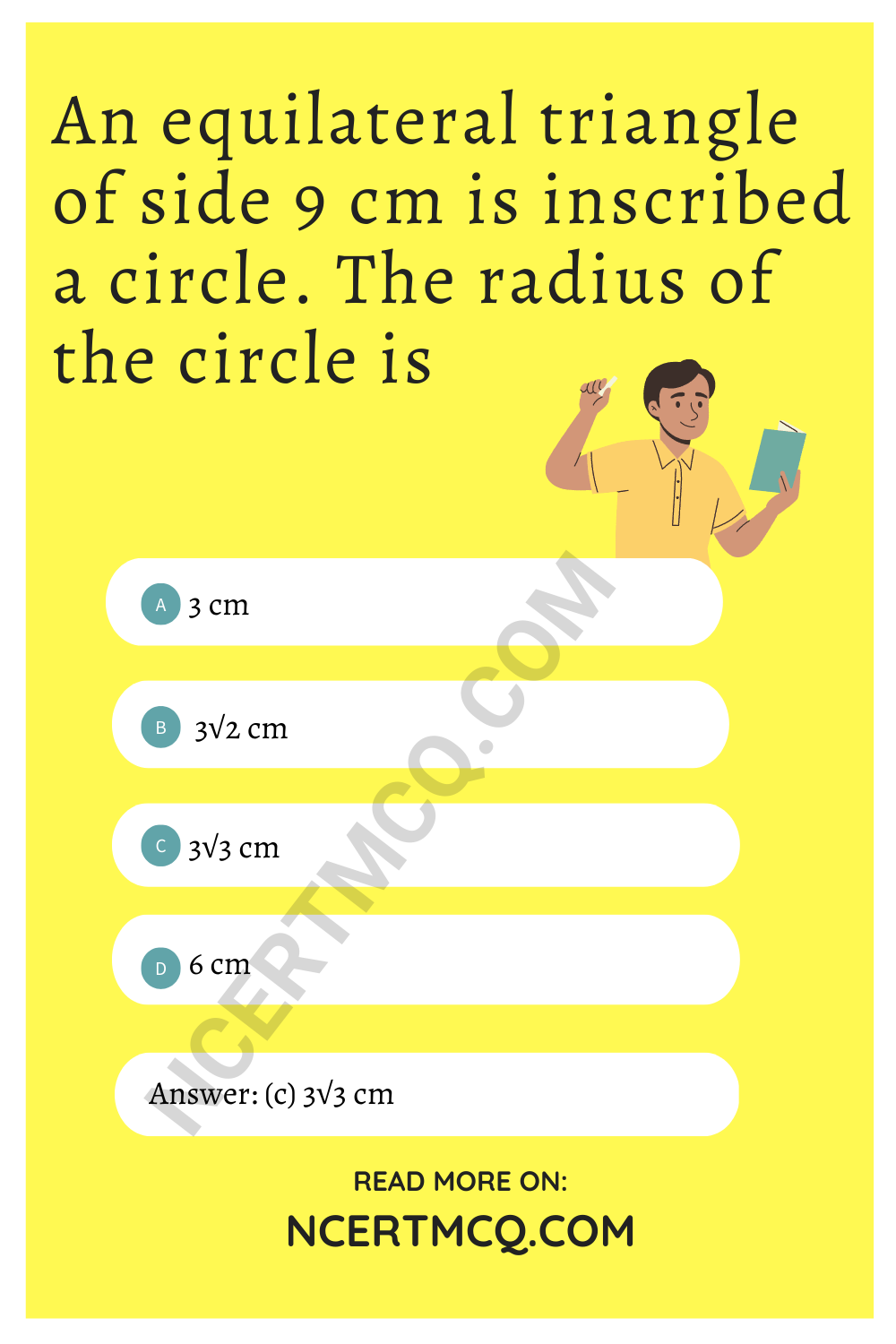MCQ Of Circles Class 9 Chapter 10 Question 10.
In the given figure, ΔABC and ΔDBC are inscribed in a circle such that ∠BAC = 60° and ∠DBC = 50°. then, ∠BCD =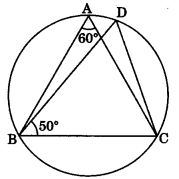(a) 50°
(b) 60°
(c) 70°
(d) 80°

MCQ Questions For Class 9 Maths With Answers Pdf Question 11.
In the given figure, O is the centre of a circle. If ∠AOB = =100° and ∠AOC = 90°, then ∠BAC =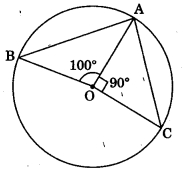(a) 85°
(b) 80°
(c) 90°
(d) 75°

Class 9 Chapter 10 MCQ Question 12.
In the given figure, O is the centre of a circle and ∠AOC = 120°. Then, ∠BDC =(a) 60°
(b) 45°
(c) 30°
(d) 15°

MCQ Questions For Class 9 Maths Chapter 10 Question 13.
In the given figure, O is the centre of a circle in which ∠OAB = 20° and ∠OCB = 50°. Then, ∠AOC =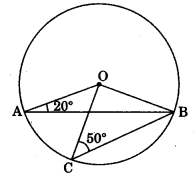(a) 50°
(b) 70°
(c) 20°
(d) 60°

Circles Class 9 MCQ Online Test Question 14.
In the given figure, O is the centre of a circle in which ∠AOC = 100° side AB of quadrilateral OABC has been produced to D. Then, ∠CBD =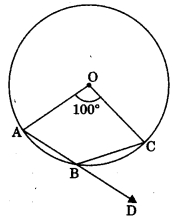(а) 50°
(b) 40°
(c) 25°
(d) 80°

Class 9 Maths Ch 10 MCQ Question 15.
In the given figure, O is the centre of a circle and ∠OAB = 50°. Then, ∠BOD =(а) 130°
(b) 50°
(c) 100°
(d) 80°

Circles Class 9 MCQ With Answers Question 16.
In the given figure, ABCD is the cyclic quadrilateral in which BC = CD and ∠CBD = 35°. Then, ∠BAD =(а) 65°
(b) 70°
(c) 110°
(d) 90°

MCQ Questions For Class 9 Maths With Answers Question 17.
In figure, ΔABC is an equilateral triangle and ABDC is a quadrilateral then ∠BDC =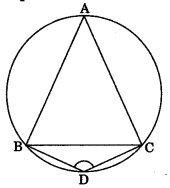(а) 90°
(b) 60°
(c) 120°
(d) 150°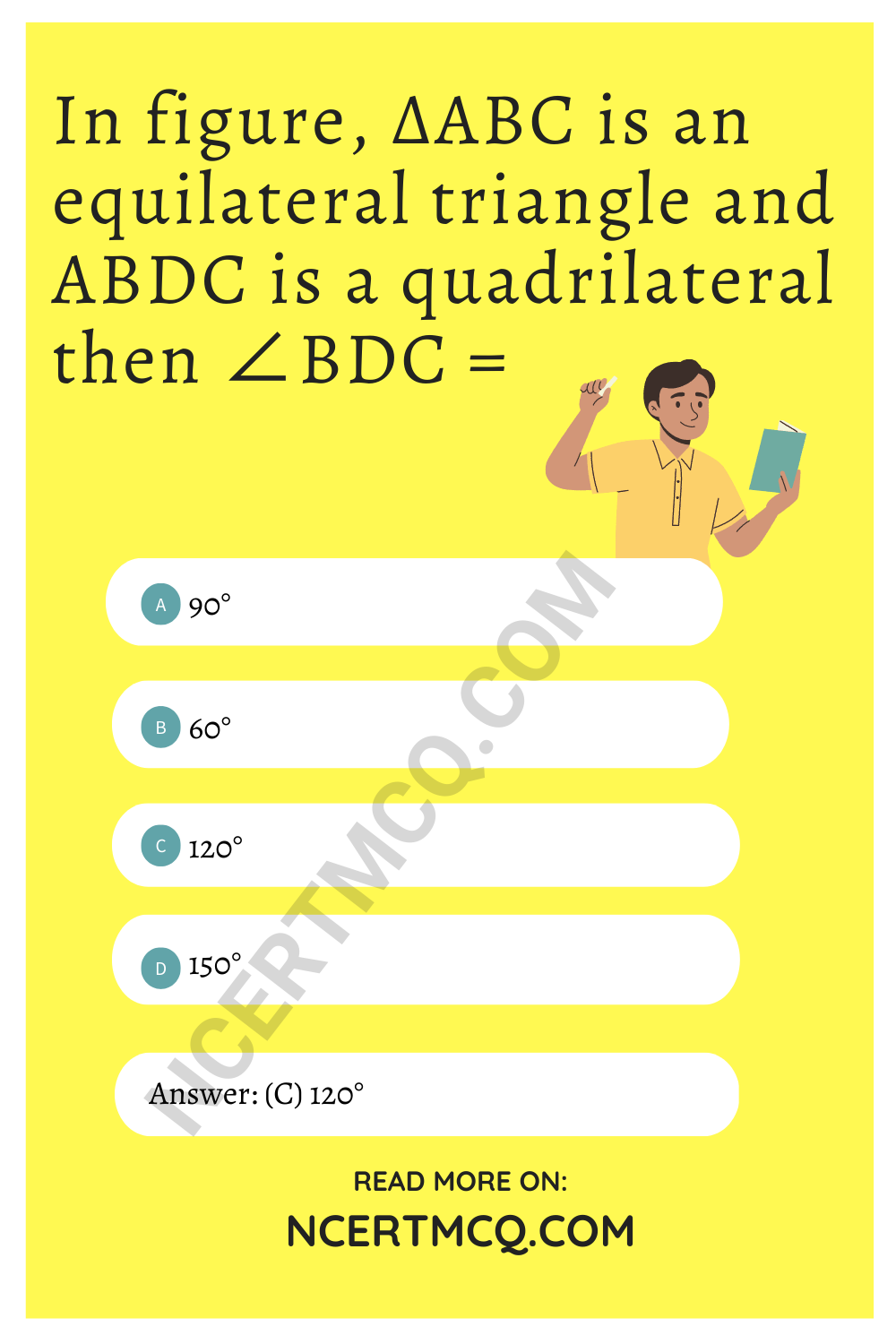Class 9 Maths Circles MCQ Question 18.
In figure, sides AB and AD of a quadrilateral ABCD are produced to E and F respectively. If ∠CBE = 100°, then find ∠CDF.(a) 100°
(b) 80°
(c) 130°
(d) 90°

MCQ Questions For Class 9 Maths Ncert Chapter 10 Question 19.
In figure, O is the centre of a circle and ∠AOB = 140°, then ∠ACB =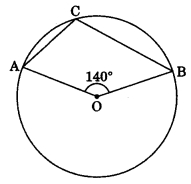(a) 70°
(b) 80°
(c) 110°
(d) 40°

Ch 10 Maths Class 9 MCQ Question 20.
In figure, O is the centre of a circle and ∠AOB = 130°, then ∠ACB =(a) 50°
(b) 65°
(c) 115°
(d) 155°